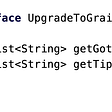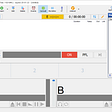# How numbers are represented in memory?

On computer systems, a byte consists of eight smaller units called bits. A bit can assume either of two values: 1 or 0.

The rightmost bit of a byte is known as the least significant bit (LSB), whereas the leftmost bit is known as the most significant bit (MSB).

Data types are of fixed width.

An integer takes 4 bytes, which is 32 bits. The rightmost bit of the byte represents 2⁰ or 1, the bit immediately to its left represents 2¹ or 2, the next bit 2² or 4, and so on.

· An unsigned integer can represent any integer from 0 to 2³²-1.

· A signed integer can represent any integer from −(2³¹) to (2³¹−1)).

Representation of positive integer

For instance ‘6’ is

· 0000 0110 (0x2³ + 1x2² + 1x2¹ + 0x2⁰) if the data type is char (1 byte)

· 0000 0000 0000 0110 if short (2 bytes)

· 0000 0000 0000 0000 0000 0000 0000 0110 if int (4 bytes)

For clarity sake I’ll use the 4-bit format.

How do we represent a negative number?

On computer systems, there’s no separate symbol that can be used as a minus sign, it’s all 0s and 1s.

For signed binary numbers the most significant bit (MSB) is used as the sign bit. If the sign bit is ‘0’, this means the number is positive in value. If the sign bit is ‘1', then the number is negative in value.

The remaining bits in the number are used to represent the magnitude of the binary number in the usual unsigned binary number format way.

With this representation, the range of a 4-bit format is from −(2³-1) to (2³-1). Which make 14 numbers out of 16 possibilities (2⁴). And there are two representations of 0: the obvious one as 0000, and the ‘negative zero’ 1000.

In a 4-bit format, ‘6’ in a signed binary would be 0110, just like in an unsigned format, and ‘-6’ would be 1110.

What if we try to add ‘6’ and ‘-6’?

As you can see, with the current representation of negative signed, the result of this operation is obviously not correct.

One’s complement

Here comes the first complement (as in two’s complements).

To get the one’s complement of a negative binary number, the bits of the positive binary number are inverted, or “flipped”, by using the bit-wise NOT operation.

So, to convert from ‘6’ (0110 in 4-bit format) to ‘-6’ in one’s complement notation, the bits are inverted, that is: ‘0’ becomes ‘1’ and ‘1’ becomes ‘0’: 1001.

If we try the previous addition of 6 + (-6) we’ve got the right result (negative zero) with this new representation of negative numbers.

But the results are not quite right with other cases: we are one off from the expected values.

As you can see there’s a pattern in all the examples here, if you add 1 to all of them, you have the right results.

Two’s complement

That’s the second’s complement: add 1.

Most computers represent negative numbers using the “two’s complement” notation.

Two’s complement has several advantages. For example, it doesn’t have the concept of ‘negative zero’. And since the ‘negative zero’ has disappeared, it makes room for an extra number in negative range, so, in a 4-bit format, the magnitude range is from −(2³) to (2³−1) instead of −(2³-1) to (2³−1) previously.

Furthermore, addition, multiplication and subtraction work the same with signed integers implemented with two’s complemented as they do with unsigned integers.

Two’s complement representation of signed integers make it easier to manipulate in hardware. Performing the same operation in raw binary (e.g. with a sign bit) usually involves a lot more work, because you must treat certain bits as special.

It does lessen the design effort and complexity of the device, thus presumably making it cheaper.

Overflow

The rules for detecting overflow in a two’s complement sum are simple:

. If the sum of two positive numbers yields a negative result, the sum has overflowed.

. If the sum of two negative numbers yields a positive result, the sum has overflowed.

That’s all, folks!

--

--

--

## More from Huy Nguyen

Cloud Data Enginneer

Love podcasts or audiobooks? Learn on the go with our new app.

## Atrix Anew## Grafana Dashboards for Windows Servers## How to Benefit from Grails 4 Upgrade## Installing mAirlist radio playout software## Architecting large-scale web applications in Flask## Angel Eyes — safer monitoring for newborns👼## Huy Nguyen

Cloud Data Enginneer

## Review of Nostalgia Ultra## Five Secrets Every Experienced Auto Accident Attorney Should Be Aware Of